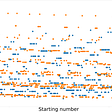# The Area of a Lune

## Another No-Algebra Geometry Puzzle

This one comes from , who in turn got it from a dead mathematician with a beard. You can check out Penn’s solution . The solution below is my own.

Figure 1 shows a right triangle parachuting to the ground with a bedsheet.

--

--

--

Maths is like finger-painting. Messy and beautiful.

## THE RELATIONSHIP BETWEEN TANGO AND MATHEMATICAL MODELS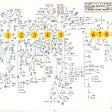## The curse of modeling detailed chemistry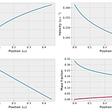## The Fundamental Theorem of Arithmetic — Solving Problems in Number Theory## python calculus## Inverse-Square Law of Gravitation: The Role of the Apple and the Moon## Probabilities. Permutations, variations, combinations.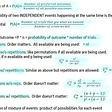## A Practical Math Problem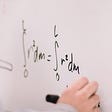## Math News — A Scale of Emotions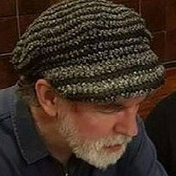Canadian math guy, experimenting with fiction. Find my new scifi/fantasy serial here:

## Rediscovering One of the Most Remarkable Formulas in Mathematics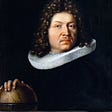## The Thrilling Story Of Calculus## Ten theorems formulated in basic-math terms proved after decades, centuries, or millennia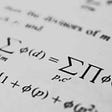## The Simplest Math Problem We Still Can’t Solve — Part 3